Friday, May 7, 2021
Home > CBSE Class 12 > NCERT Solutions for Class 12 Maths Determinants Exercise 4.4

# NCERT Solutions for Class 12 Maths Determinants Exercise 4.4# NCERT Solutions for Class 12 Maths Determinants

Hi Students, Welcome to Amans Maths Blogs (AMB). In this post, you will get the NCERT Solutions for Class 12 Maths Determinants Exercise 4.4. This NCERT Solutions can be downloaded in PDF file. The downloading link is given at last.

NCERT Solutions for Class 12 Maths are not only the solutions of Maths exercise but it builds your foundation of other important subjects. Getting knowledge of depth concept of CBSE Class 12th Maths like Algebra, Calculus, Trigonometry, Coordinate Geometry help you to understand the concept of Physics and Physical Chemistry.

CBSE Class 12th is an important school class in your life as you take some serious decision about your career. And out of all subjects, Maths is an important and core subjects. So CBSE NCERT Solutions for Class 12th Maths is major role in your exam preparation as it has detailed chapter wise solutions for all exercise.

As we know that all the schools affiliated from CBSE follow the NCERT books for all subjects. You can check the CBSE NCERT Syllabus. Thus, NCERT Solutions helps the students to solve the exercise questions as given in NCERT Books.

## NCERT Solutions for Class 12 Maths Determinants Exercise 4.4

Write Minors and Cofactors of the elements of following determinants

NCERT Solutions for Class 12 Maths Determinants Exercise 4.4: Ques No 1.

(i)NCERT Solutions: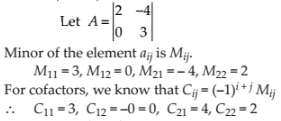(ii)NCERT Solutions: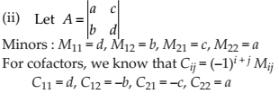NCERT Solutions for Class 12 Maths Determinants Exercise 4.4: Ques No 2.

(i)NCERT Solutions:(ii)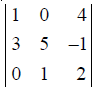NCERT Solutions: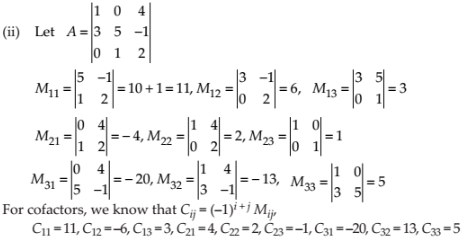NCERT Solutions for Class 12 Maths Determinants Exercise 4.4: Ques No 3.

Using Cofactors of elements of second row, evaluate Δ =NCERT Solutions: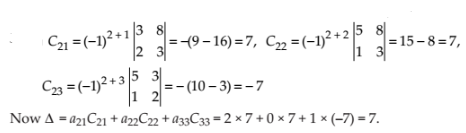NCERT Solutions for Class 12 Maths Determinants Exercise 4.4: Ques No 4.

Using Cofactors of elements of third column, evaluate Δ =NCERT Solutions:NCERT Solutions for Class 12 Maths Determinants Exercise 4.4: Ques No 5.

If Δ =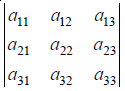and Aij is Cofactors of aij, then value of Δ is given by

(A) a11A31+ a12A32 + a13A33

(B) a11A11+ a12A21 + a13A31

(C) a21A11+ a22A12 + a23A13

(D) a11A11+ a21A21 + a31A31

NCERT Solutions:

error: Content is protected !!